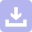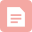# matlab向量乘法要加.,matlab中的矩阵与向量运算

4.1 数组运算和矩阵运算

4.1.1 数组运算和矩阵运算指令形式和实质内涵

A.'

A'

A=s

s+B

s-B, B-s

s.*A

s*A

s./B, B.\s

s*inv(B)

A.^n

A^n

A为方阵时,矩阵A的n次方

A+B

A+B

A-B

A-B

A.*B

A*B

A./B

A的元素被B的对应元素除

A/B

A右除B

B.\A

B\A

A左除B(一般与右除不同)

exp(A)

expm(A)

A的矩阵指数函数

log(A)

logm(A)

A的矩阵对数函数

sqrt(A)

sqrtm(A)

A的矩阵平方函数

4.2 数组的基本运算

4.2.1 点转置和共轭转置

. ' —— 点转置.非共轭转置,相当于conj(A').

>> a=1:5;

>> b=a. '

b =

1

2

3

4

5

>> c=b. '

c =

1 2 3 4 5

' —— 共轭转置.对向量进行转置运算并对每个元素取其共轭.如:

>> d=a+i*a

d =

Columns 1 through 3

1.0000 + 1.0000i 2.0000 + 2.0000i 3.0000 + 3.0000i

Columns 4 through 5

4.0000 + 4.0000i 5.0000 + 5.0000i

>> e=d'

e =

1.0000 - 1.0000i

2.0000 - 2.0000i

3.0000 - 3.0000i

4.0000 - 4.0000i

5.0000 - 5.0000i

4.2.2 纯量 (标量) 和数组的四则运算

>> g=[1 2 3 4

5 6 7 8

9 10 11 12]

>> g=g-2

g =

-1 0 1 2

3 4 5 6

7 8 9 10

>> 2*g-1

ans =

-3 -1 1 3

5 7 9 11

13 15 17 19

4.2.3 数组间的四则运算

1. 数组按元素相加,减

>> g=[1 2 3 4

5 6 7 8

9 10 11 12]

>> h=[1 1 1 1; 2 2 2 2; 3 3 3 3]

>> g+h % 按元素相加

ans =

2 3 4 5

7 8 9 10

12 13 14 15

>> ans-h % 按元素相减

ans =

1 2 3 4

5 6 7 8

9 10 11 12

>> 2*g-h % 混合运算

ans =

1 3 5 7

8 10 12 14

15 17 19 21

2. 按元素乘

>> g.*h

ans =

1 2 3 4

10 12 14 16

27 30 33 36

3. 按元素除

a./b=b.\a

>> g./h

ans =

1.0000 2.0000 3.0000 4.0000

2.5000 3.0000 4.1000 4.0000

3.0000 3.3333 3.6667 4.0000

>> h.\g

ans =

1.0000 2.0000 3.0000 4.0000

2.5000 3.0000 4.1000 4.0000

3.0000 3.3333 3.6667 4.0000

4.2.4 幂运算

>> g.^2 % 数组g每个元素的平方

ans =

1 4 9 16

25 36 49 64

81 100 121 144

>> g.^(-1) % 数组g的每个元素的倒数

ans =

1.0000 0.5000 0.3333 0.2500

0.2000 0.1667 0.1429 0.1250

0.1111 0.1000 0.0909 0.0833

>> 2.^g % 以g的每个元素为指数对2进行乘方运算

ans =

2 4 8 16

32 64 128 256

512 1024 2048 4096

>> g.^h % 以h的每个元素为指数对g中相应元素进行乘方运算

ans =

1 2 3 4

25 36 49 64

729 1000 1331 1728

>> g.^(h-1)

ans =

1 1 1 1

5 6 7 8

81 100 121 144

4.2.5 数组的指数,对数和开方运算

),log( ),sqrt( )等.

>> a=[1 3 4;2 6 5;3 2 4];

>> c=exp(a)

c =

2.7183 20.0855 54.5982

7.3891 403.4288 148.4132

20.0855 7.3891 54.5982

>>

4.3 向量运算

4.3.1 向量的点积运算

C=dot(A,B) —— 返回向量A与B的点积,结果存放于C中.

C=dot(A,B, DIM) —— 返回向量A与B在维数为DIM的点积,结果存放于C中.

>> A=[2 4 5 3 1];

>> B=[3 8 10 12 13];

>> C=dot(A,B)

C =

137

>> C=dot(A,B,4)

C =

6 32 50 36 13

4.3.2 向量的叉积运算

C=cross(A,B) —— 返回向量A与B的叉积,即:,结果存放于C中.

C=cross(A,B, DIM) —— 返回向量A与B在维数为DIM的叉积,结果存放于C中.

>> A=[2 4 5];

>> B=[3 8 10];

>> C=cross(A,B)

C =

0 -5 4

4.3.3 向量的混合运算

>> D=dot(A, cross(B,C))

D =

41

4.4 矩阵的基本运算

4.4.1 矩阵的四则运算

1. 矩阵的加减

>> a=[1 2; 3 5; 2 6];

>> b=[2 4; 1 8; 9 0];

>> c=a+b

c =

3 6

4 13

11 6

2. 矩阵的相乘

>> a=[1 2; 3 5; 2 6];

>> b=[2 4 1; 8 9 0];

>> c=a*b

c =

18 22 1

46 57 3

52 62 2

(右矩阵) 的行数时,两个矩阵的乘积才有意义.

3. 矩阵的除法

(矩阵A的秩)个非零元素,而且求得的解是这种类型的解中范数最小的一个.

>> a=[21 34 20; 5 78 20; 21 14 17; 34 31 38];

>> b=[10 20 30 40]';

>> x=b\a

x =

0.7667 1.1867 0.8767

>> a=[21 34 20 5; 78 20 21 14; 17 34 31 38];

>> b=[10 20 30]';

>> x=b\a

x =

1.6286 1.2571 1.1071 1.0500

4. 矩阵与标量间的四则运算

5. 矩阵的幂运算

>> b=[21 34 20; 78 20 21; 17 34 31];

>> c=b^2

c =

3433 2074 1754

3555 3766 2631

3536 2312 2015

6. 矩阵的指数,对数运算与开方运算

expm, expm1, expm2, expm3 —— 指数运算函数;

logm —— 对数运算函数;

sqrtm —— 开方运算函数.

>> a=[1 3 4; 2 6 5; 3 2 4];

>> c=expm(a)

c =

1.0e+004 *

0.4668 0.7694 0.9200

0.7919 1.3065 1.5613

0.4807 0.7919 0.9475

>> c=logm(a)

c =

0.5002 + 2.4406i 0.5960 - 0.6800i 0.7881 - 1.2493i

0.4148 + 0.4498i 1.4660 - 0.1253i 1.0108 - 0.2302i

0.5780 - 1.6143i 0.4148 + 0.4498i 1.0783 + 0.8263i

>> c=sqrtm(a)

c =

0.6190 + 0.8121i 0.8128 - 0.2263i 1.1623 - 0.4157i

0.3347 + 0.1497i 2.3022 - 0.0417i 1.1475 - 0.0766i

1.0271 - 0.5372i 0.3347 + 0.1497i 1.6461 + 0.2750i

7. 矩阵的转置,逆运算与行列式运算

>> a=[1 2 0; 2 5 -1; 4 10 -1];

>> c=a'

c =

1 2 4

2 5 10

0 -1 -1

>> b=inv(a)

b =

5 2 -2

-2 -1 1

0 -2 1

>> d=det(a)

d =

1

4.5 矩阵的特殊运算

4.5.1 矩阵的特征值运算

)"计算即可得到.其使用格式如下.

E=eig(X) —— 生成由矩阵X的特征值所组成的一个列向量;

[V,D]=eig(X) ——

eigs( )函数使用迭代法求解矩阵的特征值和特征向量.

D=eigs(X) —— 生成由矩阵X的特征值所组成的一个列向量.X必然是方阵,最好是大型稀疏矩阵;

[V,D]=eigs(X) ——

>> a=[1 2 0; 2 5 -1; 4 10 -1];

[b,c]=eig(a)

b =

-0.2440 -0.9107 0.4472

-0.3333 0.3333 0.0000

-0.9107 -0.2440 0.8944

c =

3.7321 0 0

0 0.2679 0

0 0 1.0000

4.5.2 矩阵 (向量) 的范数运算

)或normest( ) 计算矩阵 (向量) 的范数.其使用格式如下.

norm(X) —— 计算矩阵 (向量) X的2-范数;

norm(X,2) —— 同上;

norm(X,1) —— 计算矩阵 (向量) X的1-范数;

norm(X,inf) —— 计算矩阵 (向量) X的无穷范数;

norm(X,'fro') —— 计算矩阵 (向量) X的Frobenius范数;

normest(X) —— 只计算矩阵 (向量)

X的2-范数;并且是2-范数的估计值,适用于计算norm(X)比较费时的情况.

>> X=hilb(4)

X =

1.0000 0.5000 0.3333 0.2500

0.5000 0.3333 0.2500 0.2000

0.3333 0.2500 0.2000 0.1667

0.2500 0.2000 0.1667 0.1429

>> norm(4)

ans =

4

>> norm(X)

ans =

1.5002

>> norm(X,2)

ans =

1.5002

>> norm(X,1)

ans =

2.0833

>> norm(X,inf)

ans =

2.0833

>> norm(X,'fro')

ans =

1.5097

>> normest(X)

ans =

1.5002

4.5.3 矩阵的条件数运算

cond(X) —— 返回矩阵X的2-范数的条件数;

cond(X, P) —— 返回矩阵X的P-范数的条件数,其中P为1,2,inf或fro;

rcond(X) ——

condest(X) —— 计算关于矩阵X的1-范数的条件数的估计值.

>> M=magic(3)

M =

8 1 6

3 5 7

4 9 2

>> H=hilb(4)

H =

1.0000 0.5000 0.3333 0.2500

0.5000 0.3333 0.2500 0.2000

0.3333 0.2500 0.2000 0.1667

0.2500 0.2000 0.1667 0.1429

>> c1=cond(M)

c1 =

4.3301

>> c2=cond(M)

c2 =

4.3301

>> c3=rcond(M)

c3 =

0.1875

>> c4=condest(M)

c4 =

5.3333

>> h1=cond(H)

h1 =

1.5514e+004

>> h2=cond(H,inf)

h2 =

2.8375e+004

>> h3=rcond(H)

h3 =

3.5242e-005

>> h4=condest(H)

h4 =

2.8375e+004

4.5.4 矩阵的秩

)来计算.

>> T=rand(6)

T =

0.9501 0.4565 0.9218 0.4103 0.1389 0.0153

0.2311 0.0185 0.7382 0.8936 0.2028 0.7468

0.6068 0.8214 0.1763 0.0579 0.1987 0.4451

0.4860 0.4447 0.4057 0.3529 0.6038 0.9318

0.8913 0.6154 0.9355 0.8132 0.2722 0.4660

0.7621 0.7919 0.9169 0.0099 0.1988 0.4186

>> r=rank(T)

r =

6

>> T1=[1 1 1; 2 2 3]

T1 =

1 1 1

2 2 3

>> r=rank(T1)

r =

2下载
matlab向量矩阵运算
08-29博客
matlab矩阵对某一列求和,将矩阵的每一列另一列的对应行相，然后在Matlab求和...
03-161506博客
MATLAB的转置
10-182万+博客
Matlab 矩阵向量的基本运算
10-131万+博客
Matlab向量、点积、叉计算

06-123万+博客
【转载】Matlab基本用法小结
09-16143博客
matlab矩阵转置.'和'的不同
04-086039博客
Matlab向量矩阵

02-181088博客
matlab编程之矩阵向量的加减除操作
06-202243博客

04-129531博客
matlab计算两向量积,matlab两个函数相
03-263221博客
matlab向量,matlab如何使得两个向量对应项相
03-183762博客
matlab矩阵怎么进行相加,matlab矩阵如何运算相加
03-181万+博客
matlab矩阵合并及相关运算
07-033463博客
matlab矩阵矩阵所有元素构成的向量间相互转化
12-07329博客
matlab 向量的基本运算
01-102万+博客

03-231万+博客
matlab向量x,如何在MATLAB向量矩阵进行分量明智的乘法
03-16394博客
matlab关于矩阵向量的常用运算
12-241876

### “相关推荐”对你有帮助么？

•非常没帮助
•没帮助
•一般
•有帮助
•非常有帮助点击重新获取扫码支付余额充值